# Kamiltonian Monte Carlo (KMC) lite in unexplored regions of the target¶

1st July, 2015 by Heiko Strathmann.

This notebook illustrates that the non-adaptive version of KMC lite falls back to a random walk in unexplored regions of the target space. This in particular means that the algorithm is able to explore regions that are not part of the MCMC history after adaptation stopped.

This is in reply to Christian Robert's comment on KMC, and how a non-parametric density estimate can hurt an adaptive MCMC sampler's performance. See his book, Example 8.8. The example 8.8 uses a KDE as a proposal directly. Consequently the MCMC kernel almost never proposes in yet unexplored regions and the resulting MCMC chain converges slowly. Here we show that KMC lite does not suffer from this problem.

We set up a simple 2D Gaussian mixture, provide "oracle" samples from only one component to KMC lite (which estimates the gradient density based on those samples), and then run the algorithm without adaptation.

Disclaimer: Of course this is an easy example and there are others where the algorithm does not work that well.

In :
%load_ext autoreload

In :
import matplotlib.pyplot as plt
import seaborn as sns
%matplotlib inline

In :
import numpy as np

In :
from kmc.densities.gaussian import log_gaussian_pdf, sample_gaussian, IsotropicZeroMeanGaussian
from kmc.hamiltonian.leapfrog import leapfrog
from scripts.tools.plotting import evaluate_gradient_grid, evaluate_density_grid, plot_array
from scripts.experiments.mcmc.independent_job_classes.KMCLiteJob import KMCLiteJob

from kameleon_mcmc.tools.Visualise import Visualise


# Set up a simple 2D Gaussian mixutre¶

In :
D=2
mu1=np.zeros(D) +2
mu2=np.zeros(D) -2
Sigma1 = np.eye(D)
Sigma2 = np.eye(D)

class Posterior():
def __init__(self):
self.dimension = D

def log_pdf(self, x):
x = x.flatten()
return np.log(0.5*np.exp(log_gaussian_pdf(x, mu=mu1, Sigma=Sigma1)) +\
0.5 * np.exp(log_gaussian_pdf(x, mu=mu2, Sigma=Sigma2)))

def set_up(self):
pass

pie = Posterior()
Xs = np.linspace(-6,6)
Ys = Xs


# Oracle samples from ONE mixture component¶

In :
Z = np.vstack((sample_gaussian(N=200, mu=mu1, Sigma=Sigma1), sample_gaussian(N=200, mu=mu2, Sigma=Sigma1)))
Z = sample_gaussian(N=200, mu=mu1, Sigma=Sigma1)

Visualise.plot_density(pie, Xs, Ys)
plt.plot(Z[:,0], Z[:,1], '.');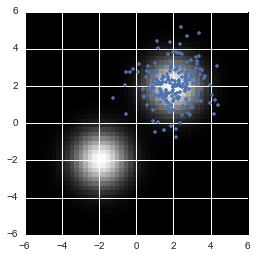## Run KMC lite with the above "oracle" samples that do not cover both modes¶

I.e. KMC never updates its density estimate, but only computes it once from the "oracle" samples. We plot the MCMC trajectory and leapfrog proposal trajectory every 100 iterations.

Note that the MCMC chain is initialised at the explored mode. We do not put special attention into tuning the hyper-parameters of the density/gradient estimator.

In :
# HMC parameters (kept simple)
step_size_min = 0.1
step_size_max = 0.1
num_steps_min = 10
num_steps_max = 10
momentum = IsotropicZeroMeanGaussian(sigma=1., D=D)

# MCMC parameters, initialise at one mode
num_iterations = 500
start = mu1

kmc_lite = KMCLiteJob(Z=Z, sigma=1., lmbda=1, target=pie, momentum=momentum, \
num_steps_min=num_steps_min, num_steps_max=num_steps_max, \
step_size_min=step_size_min, step_size_max=step_size_max, \
num_iterations=num_iterations, start=start, num_warmup=1)
kmc_lite.compute()
samples = kmc_lite.samples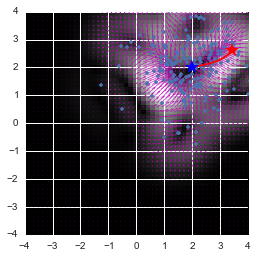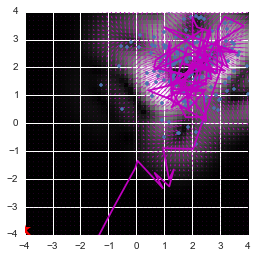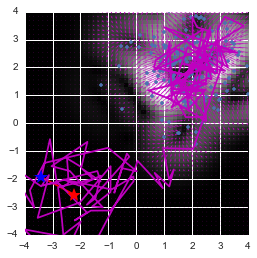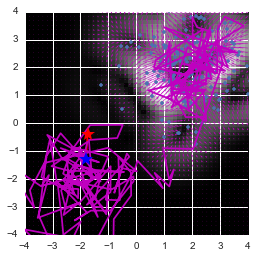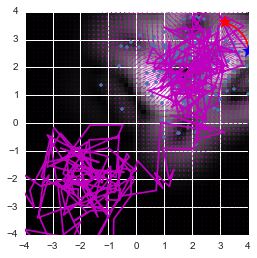The above plots show

• the norm of the gradient of the estimated kernel energy surrogate (heatmap)
• Leapfrog trajectories as red line from blue star to red star
• MCMC trajectory in magenta

Note how KMC lite eventually explores the previously unexplored mode. This is despite the fact that the density/gradient estimate never saw samples from that mode, i.e. the gradient of the estimator is effectively zero.

## The Markov chain was tuned to achieve roughly 60-70% acceptance¶

In :
np.mean(kmc_lite.accepted)

Out:
0.68400000000000005

## The joint and marginal distribution is well recovered¶

This is despite the fact that KMC lite only "saw" one mode during its exploration phase

In :
Visualise.plot_density(pie, Xs, Ys)
plt.plot(Z[:,0], Z[:,1], '.')
plt.plot(samples[:,0], samples[:,1], 'm');
plt.title("MCMC trajecory (2D)");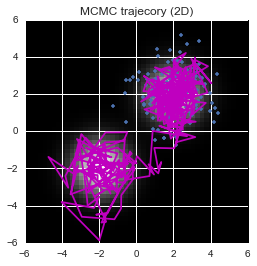In :
plt.figure(figsize=(12,5))
plt.grid(True)
plt.plot(samples[:,0], 'm');
plt.title(r"Trace $x_1$")
plt.figure(figsize=(12,5))
plt.grid(True)
plt.plot(samples[:,1], 'm');
plt.title(r"Trace $x_2$");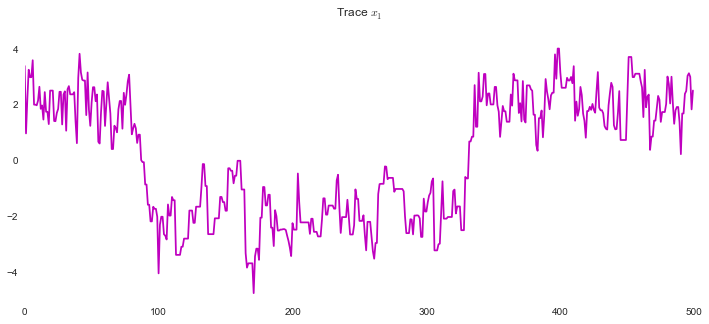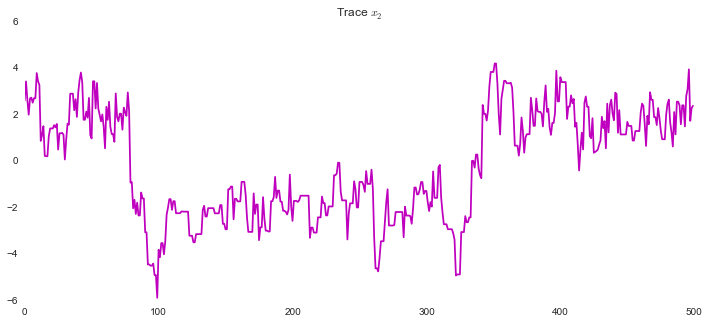In :
sns.jointplot(x=samples[:,0], y=samples[:,1], kind="kde")
sns.plt.title("Joint and marginals of MCMC trajectory (no thinning)");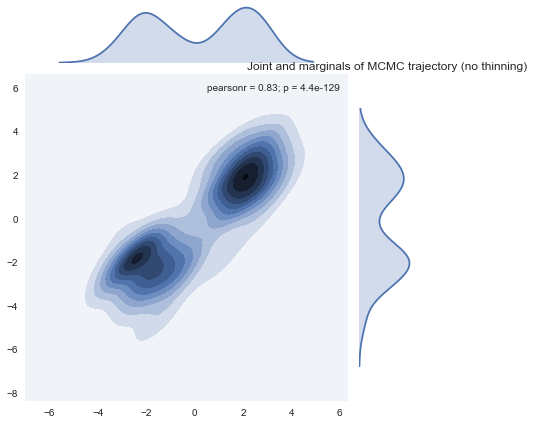## The proposals of KMC lite in unexplored regions of the MCMC chain are local¶

I.e. they follow a random walk. We plot proposals (and leapfrog trajectories) for the MCMC chain sitting at the unexplored mode.

In :
# place current point on the "unexplored" of the two modes
current = mu2

# simulate a number of proposals
for i in range(3):
# simulate the flow for some random momentum
p0 = momentum.sample()

# how does the trajectory look like?
plt.figure(figsize=(15,5))
plt.subplot(121)
plot_array(Xs, Ys, G_norm)
plt.plot(Z[:,0], Z[:,1], '.')
plt.plot(Qs[:,0], Qs[:,1], 'r-x', markersize=10)
plt.plot(current, current, 'b*', markersize=15)
plt.plot(Qs[-1,0], Qs[-1,1], 'r*', markersize=15)
plt.quiver(X, Y, U_q, V, color='m')

plt.subplot(122)
G = evaluate_density_grid(Xs, Ys, lambda x: np.exp(pie.log_pdf(x)))
plot_array(Xs, Ys, G)
plt.plot(Z[:,0], Z[:,1], '.')
plt.plot(Qs[:,0], Qs[:,1], 'r-x', markersize=10)
plt.plot(current, current, 'b*', markersize=15)
plt.plot(Qs[-1,0], Qs[-1,1], 'r*', markersize=15)
plt.title("True target density")

plt.show()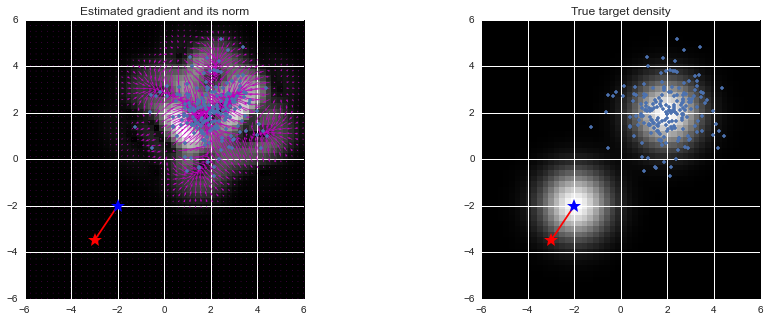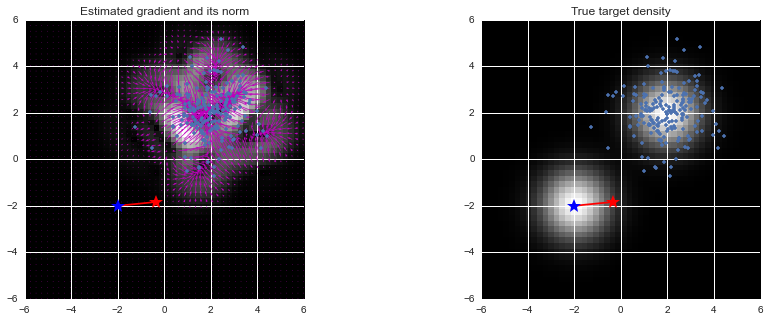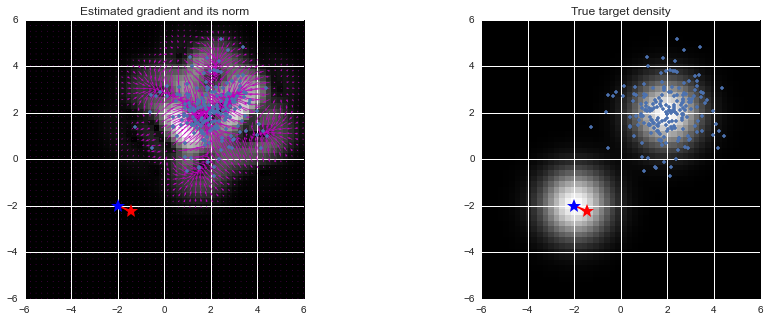# KDE proposals¶

In contrast, using a KDE of the oracle samples as a proposal as in Example 8.8 completely fails to visit the "unseen" mode.

We fit a KDE on the same samples as above (only covering one mode), and then run a simple MH-kernel with the KDE as a proposal, initialised at the "seen" mode.

In :
# fit a KDE using scikit learn
from sklearn.neighbors import KernelDensity

kde=KernelDensity(bandwidth=1.).fit(Z)

# run MCMC chain from "seen" mode
samples2 = np.zeros((num_iterations, D))
current = mu1
for i in range(num_iterations):
current_log_prob = pie.log_pdf(current)
proposal = kde.sample()
proposal_log_prob = kde.score(proposal)

acc_prob = np.min([1, np.exp( \
pie.log_pdf(proposal) + kde.score(current) \
- pie.log_pdf(current)  -kde.score(proposal)) \
])
if np.random.rand() < acc_prob:
current = proposal

samples2[i] = current

In :
plt.figure(figsize=(12,5))
plt.grid(True)
plt.plot(samples2[:,0], 'm');
plt.title(r"Trace $x_1$")
plt.figure(figsize=(12,5))
plt.grid(True)
plt.plot(samples2[:,1], 'm');
plt.title(r"Trace $x_2$");